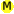Iteration

Arc provides a number of macros to iterate over code multiple times. `while`, `until`, `whilet`, `whiler`, and `loop` permit iteration subject to a condition. `drain` iterates over an expression, collecting the values. `for` and `repeat` iterate a specified number of times. `forlen`, `each`, `noisy-each`, `on`, and `ontable` iterate over a sequence.

Iterationwhile test [body ...] Executes `body` repeatedly while `test` is true. The test is evaluated before each execution of `body`. ```>(let x 0 (while (< x 3) (prn x) (++ x))) 0 1 2 nil ```until test [body ...] Executes `body` repeatedly until `test` is true. The test is evaluated before each execution of `body`. `until` is the opposite of `while`. ```>(let x 0 (until (> x 3) (prn x) (++ x))) 0 1 2 3 nil ```whilet var test [body ...] Executes `body` repeatedly while `test` is true. The value of `test` is assigned to `var` on each iteration, for use in the body. Typically `test` obtains a new value from some source, and whilet is used to loop until `nil` is obtained. ```>(w/instring s "abc" (whilet c (readc s) (prn c))) a b c nil ```whiler var expr endval [body ...] Executes `body` repeatedly while `expr` is not `endval`. The value of `expr` is assigned to `var` on each iteration. ```>(w/instring s "abcdef" (whiler x (readc s) #\d (prn x))) a b c nil ```loop start test update [body ...] Executes `start`, then executes `body` repeatedly, checking `test` before each iteration and executing `update` afterward. ```>(loop (= x 0) (< x 3) (++ x) (prn x)) 0 1 2 nil ```drain expr [eof] Repeatedly executes `expr` until it returns `eof` (default `nil`). A list of the `expr` values is returned. ```>(w/instring s "(1 2) (3 4)" (drain (sread s nil))) ((1 2) (3 4)) ``` ```>(let x 256 (drain (= x (/ x 2)) 1)) (128 64 32 16 8 4 2) ```for var init max [body ...] Executes `body` repeatedly with `var` assigned the values from `init` to `max`, incremented by 1 each time. For the last iteration, `v` will be >= `max`. If `max <= init-1`, `body` will not be executed. ```>(for x 2.5 4.0 (prn x)) 2.5 3.5 4.5 nil ``` ```>(for x 2.5 1.6 (prn x)) 2.5 nil ```repeat n [body ...] Executes `body` `n` times. Non-integers are rounded up. ```>(repeat 3 (prn "hi")) hi hi hi nil ```forlen var seq [body ...] Iterates over a sequence (list, string, or table) `seq`. `var` takes the values from 0 to `length`-1. ```>(let seq '(1 2 3) (forlen x seq (prn x " " (seq x)))) 0 1 1 2 2 3 nil ``` ```>(let seq "abc" (forlen x seq (prn x " " (seq x)))) 0 a 1 b 2 c nil ``` ```>(let seq (obj 0 'val0 1 'val1) (forlen x seq (prn x " " (seq x)))) 0 val0 1 val1 nil ```each var expr [body ...] Executes `body`, with `var` taking on each value from `expr`, which can be a list, string, or table. For a table, `var` takes on the values, not the keys. ```>(each x '(1 (2 3) 4) (prn x)) 1 (2 3) 4 nil ``` ```>(each x "abc" (prn x)) a b c nil ``` ```>(each x (obj key1 'val1 key2 'val2) (prn x)) (key1 val1) (key2 val2) #hash((key1 . val1) (key2 . val2)) ```noisy-each n var val [body ...] Version of `each`, printing a dot every `n` iterations. ```>(noisy-each 3 x "abcdefghijk" (pr x)) ab.cde.fgh.ijk t ```on var s [body ...] Iterates the same as `each`, except the variable `index` is assigned a count, starting at 0. For tables, `var` is assigned `nil` each iteration, so `ontable` is probably more useful. ```>(on x '(1 (2 3) 4) (prn index " " x)) 0 1 1 (2 3) 2 4 nil ``` ```>(on x "abc" (prn index " " x)) 0 a 1 b 2 c nil ``` ```>(on x (obj key1 'val1 key2 'val2) (prn index " " x)) 0 nil 1 nil nil ```ontable k v tab [body ...] Iterates over the table `tab`, assigning `k` and `v` each key and value. ```>(ontable k v (obj key1 'val1 key2 'val2) (prn k " " v)) Error: _ontable: undefined; cannot reference an identifier before its definition in module: top-level internal name : _ontable ```